MLZ: Mean Length-based Z Estimators

1. Introduction

MLZ is a package that facilitates data preparation and estimation of mortality with statistical diagnostics using the mean length-based mortality estimator and several extensions.

2. Outline of package

In this section, a step-by-step guide to using the mean length (ML) estimator of Gedamke and Hoenig (2006) is provided. This guide outlines the main features of the package.

Work in the package can be divided into three general steps, with supporting diagnostic tools:

1. Data preparation
2. Mortality estimation
3. Model selection procedure

2.1 Data preparation

MLZ uses the S4 class system. Data and life history parameters, i.e., von Bertalanffy Linf and K, are stored in a single object of class MLZ_data with pre-defined slots. Slots in the S4 class can be accessed with @.

library(MLZ)
class?MLZ_data
data(Goosefish)
Goosefish@vbLinf

Length data are imported as either a data frame of individual records or as a matrix (years x length bins):

data(SilkSnapper)
new.dataset <- new("MLZ_data", Year = 1983:2013, Len_df = SilkSnapper, length.units = "mm")

The bin_length function can be used to convert individual lengths into a length frequency matrix with specified length bins.

bin_length(SilkSnapper)

The plot function can be used to visualize the data to aid in the selection of $$L_c$$.

plot(new.dataset, type = "comp")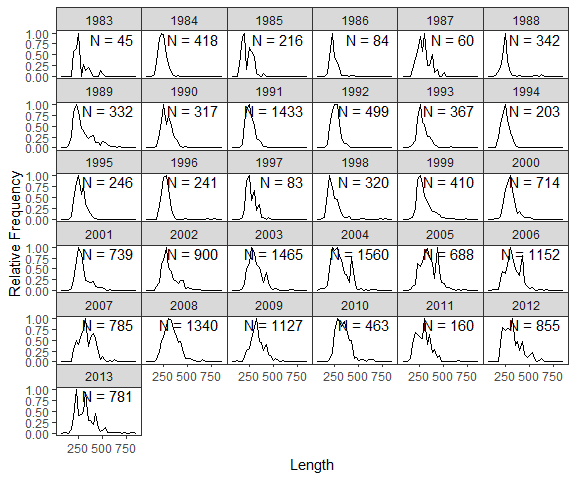Once Lc is identified, calc_ML() can be used to mean lengths from records larger than Lc.

new.dataset@Lc <- 310
new.dataset <- calc_ML(new.dataset)

new.dataset@MeanLength
new.dataset@ss

A summary method function is also available for class MLZ_data.

summary(new.dataset)

2.2 Mortality estimation

Once mean lengths > Lc are calculated, mortality can be estimated using the ML function:

est <- ML(Goosefish, ncp = 2)

The function returns an object of class MLZ_model which includes predicted values of the data, parameter estimates with correlation matrix and gradient vector. summary and plot method functions are also available for MLZ_model objects.

plot(est)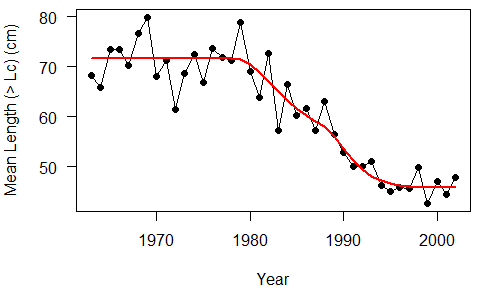summary(est)
## $Stock ##  "Goosefish: Northern Management Region" ## ##$Model
##  "ML"
##
## $Estimates ## Estimate Std. Error ## Z 0.141 0.005 ## Z 0.310 0.037 ## Z 0.551 0.046 ## yearZ 1978.316 0.832 ## yearZ 1987.767 1.222 ## sigma 24.342 0.000 With i = 1, 2, ... I change points, Z[i] is the estimated mortality rate in successive time periods. yearZ[i] indicates the time when mortality changed from Z[i] to Z[i+1]. The analysis can be repeated by considering alternative numbers of change points (years in which mortality changes, estimated in continuous time). model1 <- ML(Goosefish, ncp = 0) model2 <- ML(Goosefish, ncp = 1) model3 <- ML(Goosefish, ncp = 2) 2.3 Model selection Model runs with different change points can be compared using AIC. The compare_models function facilitates this feature and produces a plot of the predicted data. compare_models(model1, model2, model3)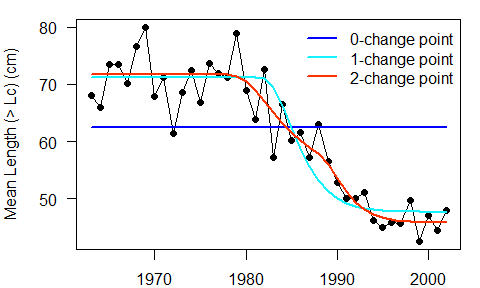## negLL npar AIC delta.AIC ## 2-change point 111.1122 6 234.2244 0.000000 ## 1-change point 116.5551 4 241.1103 6.885858 ## 0-change point 157.9197 2 319.8394 85.615006 compare_models can also be used with MLCR and MLmulti (See section 4). 3. Diagnostic tools 3.1 Data preparation To explore changes in the length distribution over time, the modal_length function plots the modal length from each annual length distribution. The modal length can change for several reasons, including changes in mortality, selectivity, and recruitment. modal_length(new.dataset, breaks = seq(80, 830, 10))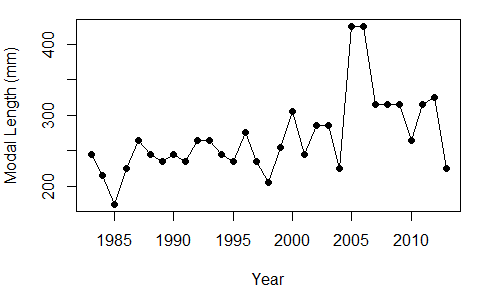3.2 Mortality estimation 3.2.1 Grid search & likelihood profile of change points In order to avoid local minima in the negative log-likelihood function, the estimation functions by default use a grid search over the change points in order to find starting values close to the maximum likelihood estimates. The grid search function can also be called seperately using profile_ML. This function also serves as a likelihood profile over the change points. Figures are provided for 1- and 2-change point models. profile_ML(Goosefish, ncp = 1)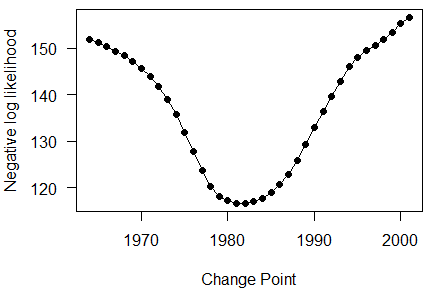profile_ML(Goosefish, ncp = 2)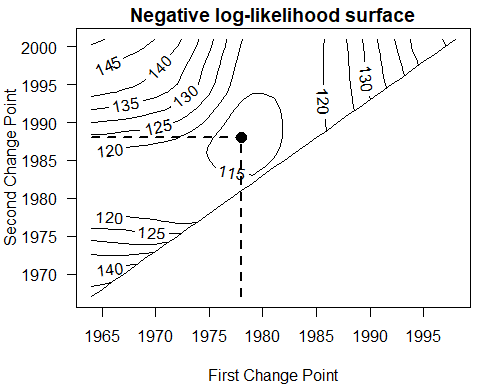profile_MLCR, and profile_MLmulti are also available for the respective models (Section 4). 3.2.2 Sensitivity to Lc The sensitivity_Lc function for the ML estimator plots estimates of Z and change points with different values of Lc. 3.2.3 Additional diagnostics Additional diagnostics, including sensitivity to life hitory (growth, natural mortality), and bootstrapping routines are in development. 4. Outline of other estimators 4.1 MLCR (Mean Length with Catch Rate) To use the MLCR estimator (Huynh et al. 2017b), a time series of CPUE is needed: data(MuttonSnapper) MuttonSnapper@CPUE If the CPUE is biomass-based, e.g., pounds of fish per gear haul, then length-weight exponent b is also needed. MuttonSnapper@lwb <- 3.05 The corresponding estimation function and grid search function are MLCR and profile_MLCR, respectively: MLCR(MuttonSnapper, ncp = 2, "WPUE") 4.2 MLmulti (Multispecies Mean Length estimator) For a multispecies analysis (Huynh et al. 2017a), seperate MLZ_data objects are created for seperate stocks/species and should be stored in a list: data(PRSnapper) typeof(PRSnapper) ##  "list" The corresponding estimation function and grid search function are MLmulti and profile_MLmulti, respectively. For both functions, the Single Species Model or Multispecies 1, 2, or 3 must also be identified in the model argument: MLmulti(PRSnapper, ncp = 1, model = "MSM1") In component estimates of the output object, the mortality estimates Z[i,n] are indexed by time period i and species n, change points yearZ[i] are indexed by time period i, and sigma[n] is indexed by species n. For models MSM2 and MSM3, Z[i,n] are estimated for i = 1 and derived for i > 1. Esimated parameters can be viewed by checking component opt from the output: res <- MLmulti(PRSnapper, ncp = 1, model = "MSM1") names(res@opt$par)

The compare_models function will correctly count the number of estimated parameters for AIC calculation.

4.3 MLeffort (Mean Length with Effort)

MLeffort uses a time series of mean length and effort to estimate a catchability coefficient q and natural mortality M (Then et al.). Parameter $$t_0$$ from the von Bertalanffy equation is needed as well:

data(Nephrops)
Nephrops@Effort
Nephrops@vbt0 <- 0
MLeffort(Nephrops, start = list(q = 0.1, M = 0.2), n_age = 24)

Unlike other models in the package, starting values are required in MLeffort.

Instead of using an analytic model for the mean length, MLeffort uses an age-structured population model. The youngest age in the age-structured model is $$t_c$$ which is obtained from von Bertalanffy parameters and $$L_c$$: $$t_c = \frac{-1}{K}log(1 - \frac{L_c}{L_{\infty}}) + t_0$$

In the MLeffort function call, the number of ages above $$t_c$$ to be modeled is specified in argument n_age. Time steps smaller than one year can be used by indicating the number of seasons in the model with argument n_season. The season is matched to the season in which mean lengths are observed with argument obs_season. Currently only one observation per year is supported. The timing within the observed season that lengths are observed is set with argument timing, i.e. timing = 0, 0.5 is the beginning and middle of the season, respectively. The equilibrium effort prior to the first year of the model is indicated with argument eff_init, i.e. eff_init = 0 for virgin equilibrium conditions.

MLeffort(Nephrops, start = list(q = 0.1, M = 0.3), n_age = 24, n_season = 1, obs_season = 1, timing = 0.5)

Finally, the model can be run with a fixed M with the argument estimate.M = FALSE, in which case, the value of M for the model is obtained from slot @M in the MLZ_data object.

Nephrops@M <- 0.3
MLeffort(Nephrops, start = list(q = 0.1), n_age = 24, estimate.M = FALSE)